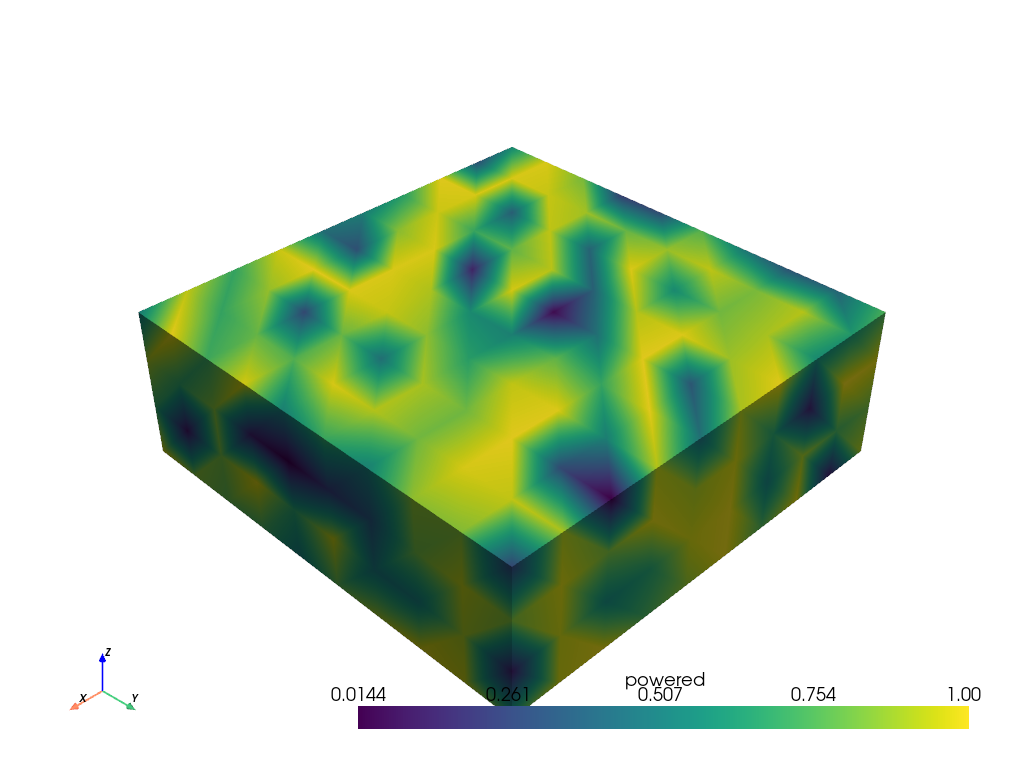# Array Math¶

This example will demonstrate how to perform a mathematical operation between two input arrays for any given source.

This filter allows the user to select two input data arrays on which to perform math operations. The input arrays are used in their order of selection for the operations.

This example demos: `PVGeo.filters.ArrayMath`

```import numpy as np
import pyvista
import PVGeo
from PVGeo.filters import ArrayMath
```

Create some input data. This can be any vtkDataObject

```inp = pyvista.UniformGrid((10,10,4))
# Populate the tables
n = 400
arr0 = np.random.random(n)
arr1 = np.random.random(n)
inp['Array 0'] = arr0
inp['Array 1'] = arr1
```

Use the filter:

```f = ArrayMath(operation='add', new_name='foo')
# Now get the result
output = f.apply(inp, 'Array 0', 'Array 1')
print(output)
```

Out:

```UniformGrid (0x7f4faadda8e8)
N Cells:      243
N Points:     400
X Bounds:     0.000e+00, 9.000e+00
Y Bounds:     0.000e+00, 9.000e+00
Z Bounds:     0.000e+00, 3.000e+00
Dimensions:   10, 10, 4
Spacing:      1.000e+00, 1.000e+00, 1.000e+00
N Arrays:     3
```

Note that the output now has three arrays

```arr = output['foo']
assert(np.allclose(arr, arr0+arr1))
```

Use a custom math operation:

```def power(arr0, arr1):
return arr0**arr1

# Use filter generated above
f.set_operation(power)
f.set_new_array_name('powered')
f.update()

# Now get the result
output = f.get_output()
print(output)
```

Out:

```UniformGrid (0x7f4faadda7c8)
N Cells:      243
N Points:     400
X Bounds:     0.000e+00, 9.000e+00
Y Bounds:     0.000e+00, 9.000e+00
Z Bounds:     0.000e+00, 3.000e+00
Dimensions:   10, 10, 4
Spacing:      1.000e+00, 1.000e+00, 1.000e+00
N Arrays:     3
```
```arr = output['powered']
assert(np.allclose(arr, arr0**arr1))
```
```output.plot(scalars='powered')
```Out:

```[(19.085160130469124, 19.085160130469124, 16.085160130469124),
(4.5, 4.5, 1.5),
(0.0, 0.0, 1.0)]
```

Total running time of the script: ( 0 minutes 0.427 seconds)

Gallery generated by Sphinx-Gallery